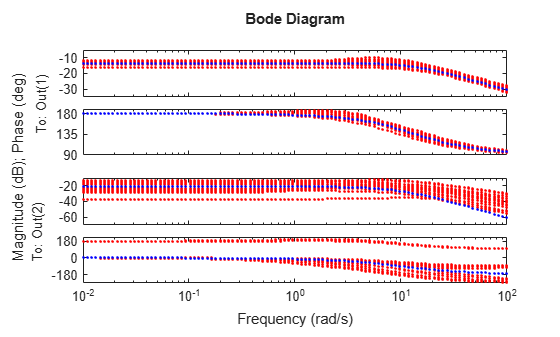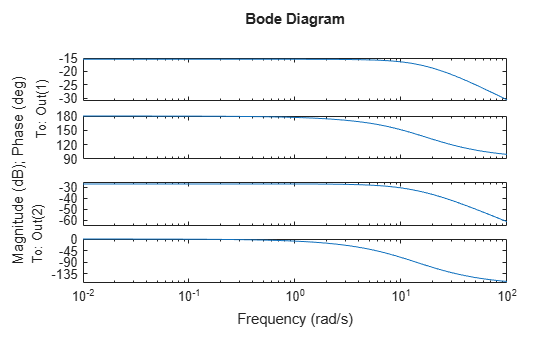# Create Uncertain Frequency Response Data Models

Uncertain frequency responses (`ufrd`) arise naturally when computing the frequency response of an uncertain state-space model (`uss`). They also arise when frequency response data in an `frd` model object is combined with an uncertain matrix (`umat`) such as by adding, multiplying, or concatenating.

To take the frequency response of an uncertain state-space model, use the `ufrd` command. Construct an uncertain state-space model.

```p1 = ureal('p1',10,'pe',50); p2 = ureal('p2',3,'plusm',[-.5 1.2]); p3 = ureal('p3',0); A = [-p1 p2;0 -p1]; B = [-p2;p2+p3]; C = [1 0;1 1-p3]; D = [0;0]; sys = ss(A,B,C,D) ```
```Uncertain continuous-time state-space model with 2 outputs, 1 inputs, 2 states. The model uncertainty consists of the following blocks: p1: Uncertain real, nominal = 10, variability = [-50,50]%, 2 occurrences p2: Uncertain real, nominal = 3, variability = [-0.5,1.2], 2 occurrences p3: Uncertain real, nominal = 0, variability = [-1,1], 2 occurrences Type "sys.NominalValue" to see the nominal value and "sys.Uncertainty" to interact with the uncertain elements. ```

Compute the uncertain frequency response of the uncertain system. Use `ufrd` command with a frequency grid of 100 points. The result is an uncertain frequency response model object, a `ufrd` model.

`sysg = ufrd(sys,logspace(-2,2,100)) `
```Uncertain continuous-time FRD model with 2 outputs, 1 inputs, 100 frequency points. p1: Uncertain real, nominal = 10, variability = [-50,50]%, 2 occurrences p2: Uncertain real, nominal = 3, variability = [-0.5,1.2], 2 occurrences p3: Uncertain real, nominal = 0, variability = [-1,1], 2 occurrences Type "sysg.NominalValue" to see the nominal value and "sysg.Uncertainty" to interact with the uncertain elements. ```

### Properties of `ufrd` Model Objects

View the properties of the model object.

`get(sysg)`
``` Frequency: [100x1 double] FrequencyUnit: 'rad/TimeUnit' ResponseData: [2x1x100x1 umat] NominalValue: [2x1 frd] Uncertainty: [1x1 struct] InputDelay: 0 OutputDelay: [2x1 double] InputName: {''} InputUnit: {''} InputGroup: [1x1 struct] OutputName: {2x1 cell} OutputUnit: {2x1 cell} OutputGroup: [1x1 struct] Notes: [0x1 string] UserData: [] Name: '' Ts: 0 TimeUnit: 'seconds' SamplingGrid: [1x1 struct] ```

The properties `ResponseData` and `Frequency` behave the same as the corresponding properties in Control System Toolbox™ `frd` objects, except that `ResponseData` is an uncertain matrix (`umat`). The properties `InputName`, `OutputName`, `InputGroup`, and `OutputGroup` behave in exactly the same manner as for all of the Control System Toolbox model objects such as `ss`, `zpk`, `tf`, and `frd`.

The `NominalValue` property is an `frd` object. Hence all functions you can use to analyze `frd` objects can also analyze `ufrd` objects. are available. When you use analysis commands such as `bode` or `step` with an uncertain model, the command plots random samples of the response to give you a sense of the variation. For instance, plot sampled Bode responses of the system along with the nominal response, using a dot marker so that you can see the individual frequency points.

`bode(sysg,'r.',sysg.NominalValue,'b.')`Just as with `umat` uncertain matrices and `uss` uncertain models, the `Uncertainty` property of the `ufrd` model is a structure containing the uncertain elements. In the model `sysg`, all uncertain elements are `ureal` parameters. Change the nominal value of the uncertain element `p1` within `sysg` to 14, and plot the Bode response of the (new) nominal system.

`sysg.Uncertainty.p1.NominalValue = 14`
```Uncertain continuous-time FRD model with 2 outputs, 1 inputs, 100 frequency points. p1: Uncertain real, nominal = 14, variability = [-50,50]%, 2 occurrences p2: Uncertain real, nominal = 3, variability = [-0.5,1.2], 2 occurrences p3: Uncertain real, nominal = 0, variability = [-1,1], 2 occurrences Type "sysg.NominalValue" to see the nominal value and "sysg.Uncertainty" to interact with the uncertain elements. ```
`bode(sysg.NominalValue)`### Lifting an `frd` model to a `ufrd` model

A non-uncertain frequency response model is equivalent to an uncertain frequency response model with no uncertain elements. Use the `ufrd` command to “lift” an `frd` model to the `ufrd` class.

```sys = rss(3,2,1); sysg = frd(sys,logspace(-2,2,100)); usysg = ufrd(sysg) ```
```Uncertain continuous-time FRD model with 2 outputs, 1 inputs, 100 frequency points, and no uncertain blocks. Type "usysg.NominalValue" to see the nominal value and "usysg.Uncertainty" to interact with the uncertain elements. ```

You can also lift arrays of `frd` objects. See Array Management for Uncertain Objects for more information about how arrays of uncertain objects are handled.